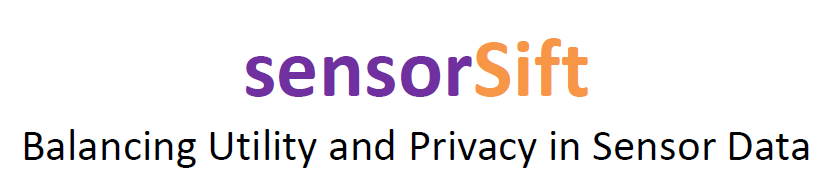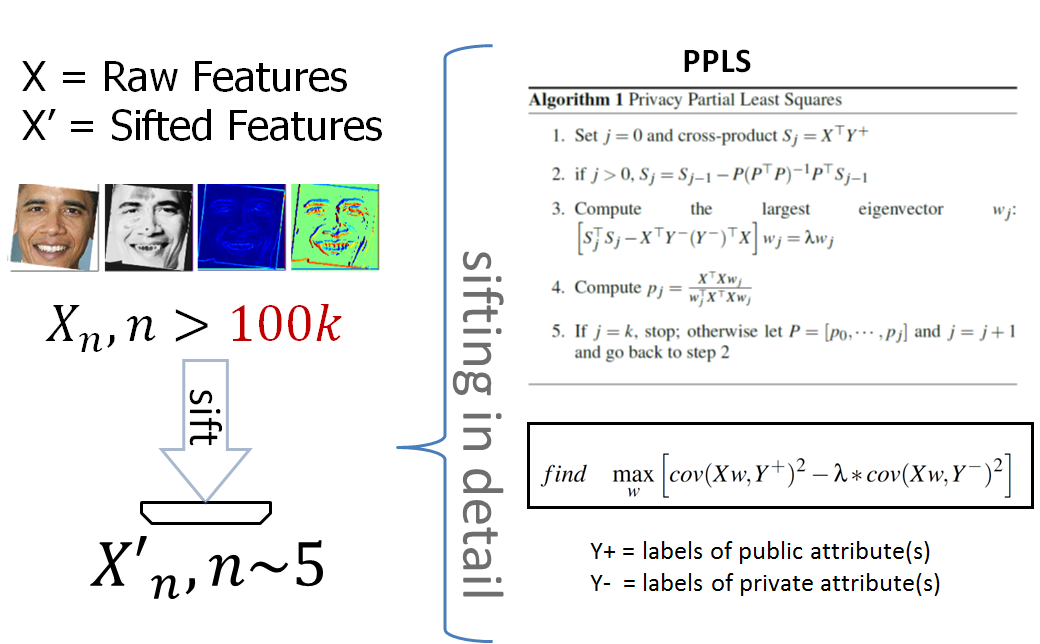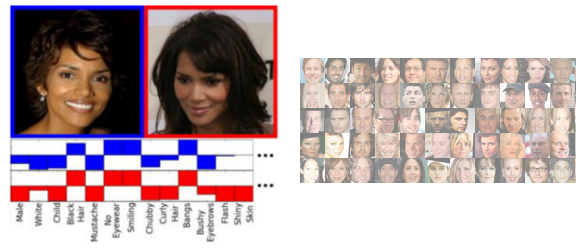The rapid growth of sensors and algorithmic reasoning are creating an important challenge to find balance between user privacy and functionality in smart applications. To address this problem Jaeyeon Jung, Liefeng Bo, Xiaofeng Ren, Tadayoshi Kohno, and I have developed a quantitative framework called SensorSift which we recently published and have now made available as open source! SensorSift is a principled framework for computing transformations (called sifts) of raw [sensor] data to enable a simultaneous balance between user's privacy choices and applications data requests. The mathematical algorithm for generating sifts is described in our ACSAC '12 paper. Below we have made the source code available under the BSD license. If you use this tool, please cite the following paper:
SensorSift: Balancing Sensor Data Privacy and Utility in Automated Face Understanding. Miro Enev, Jaeyeon Jung, Liefeng Bo, Xiaofeng Ren, Tadayoshi Kohno. Annual Computer Security Applications Conference (ACSAC), 2012
[bibtex] [pdf] [ slides ]

### Algorithm

Intuitively the sift generating algorithm finds safe region(s) in feature space which are strongly correlated with public but not private attributes. Raw data can then be projected onto these regions prior to being released to 3rd parties. More formally we introduce a novel algorithm called Privacy Partial Least Squares (PPLS), which modifies the objective function of traditional Partial Least Squares to enable for the generation of policy directed data transformations which seek to maximize the gap between public and private attribute covariances and data regions. For additional details we invite you to refer to our paper.### Code

The source code of the PPLS algorithm is available here under the BSD license. The inputs to the function are as follows: ``` X: NxD1 matrix, N is the number of raw data samples and D1 is their feature dimensionality pub_labels: NxD2 matrix, indicating the presence/absence of the D2 public attributes (via binary labels) priv_labels: NxD3 matrix, indicating the presence/absence of the D3 private attributes (via binary labels) ncomp: the maximum sift dimensionality desired (default is 10) lambda: tradeoff parameter between privacy/utility used in the objective function (default is 1) ``` The output is a sift matrix ` sift_mat ` which can be applied to raw data vectors.

#### Example Workflow:

Below is an example using the variables defined above, and a 50/50 data split between training and test data. To be explicit we introduce the following variables to track train and test indexes: train_samples = 50% of samples; test_samples = any non train samples (remaining 50%).
``` ```
``` % generate sift using training samples sift_mat = PPLS_sift_gen ( X(train_samples, :), ... pub_labels(train_samples, :), ... priv_labels(train_samples, :) ); % apply sift to test data X_sifted = X(test_samples,:) * sift_mat; % evaluate performance using ensemble of classifiers for method_num = 1:num_methods accuracy(method_num) = ML_method( method_num, X_sifted, ... pub_labels(test_samples, :), ... priv_labels(test_samples, :) ); ```

### Measuring Performance // Results

To measure the quality of a sift we use the following two metrics. In the ideal case both of these values will be minimized:
• PubLoss - how much classification accuracy is sacrificed on sifted public attribute(s) relative to raw (unsifted) data
• PrivLoss - increase in classification performance of private attributes relative to random guessing
Note that we use class average accuracy instead of aggregate accuracy in these metrics.
Class Average Accuracy is computed in the following way:
``` ClassAvgAccuracy = ( tP/(tP+fP) + tN/(tN+fN) )/2 ```
Where tP = true positives, fP = false positives, tN = true negatives, fN = false negatives.
With ClassAvgAccuracy attribute presence (positive hit rate) and attribute absence (negative hit rate) are equally weighted; this in turn emphasizes classifier precision and offers less sensitivity to attributes with imbalanced ratios of positive to negative data instances.
We measured classification accuracy using 5 modern machine learning techniques listed below:
• Neural Net (Feed Forward)
• Random Forest (Decision Trees)
• Linear Support Vector Machine
• Non-Linear Support Vector Machine
• Nearest Neighbors Clustering

For the formal definitions of sift performance (PubLoss and PrivLoss) as well as for the parameters used to define our classifiers please refer to Section 6 of our paper .

### Case StudyIn the context of our study we evaluated the sifting algorithm using face images. We show that for many policies based on facial attributes it is possible to find privacy preserving sifts which retain the classifiability of public attributes in the transformed data.
The face dataset we used for evaluation is the PubFig Face Database v 1.2 available from http://www.cs.columbia.edu/CAVE/databases/pubfig/. Note that for licensing reasons the image set is not directly available, but the image URLS themselves are in the public domain. When we retrieved these images we found that a non-trivial amount of these URLS were not accessible (~20%).

### Feedback/Contact

This project was made possible with the generous support of: